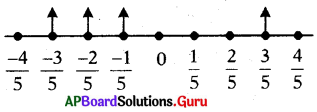SCERT AP 7th Class Maths Solutions Pdf Chapter 2 Fractions, Decimals and Rational Numbers Unit Exercise Questions and Answers.

## AP State Syllabus 7th Class Maths Solutions 2nd Lesson Fractions, Decimals and Rational Numbers Unit Exercise

Question 1.
(i) The set of integers are denoted by [ ]
(A) N
(B) W
(C) Z
(D) Q
(C) Z

(ii) Number of decimal places in the product of 48.23 × 0.2 [ ]
(A) 2
(B) 3
(C) 1
(D)5
(B) 3

(iii) Number of decimal places to the quotient of 537.1 + 10 [ ]
(A) 1
(B) 2
(C) 4
(D) 3
(B) 2

(iv) An integer can be ____________
(A) negative
(B) positive
(C) zero
(D) all the above
(D) all the above

Question 2.
Fill in the blanks:
(i) The numbers written in the form of $$\frac{p}{q}$$, where p. q are integers and q ≠ 0 are __________ numbers

(ii) 0.11 × 0.11 = __________
0.11 × 0.11
= $$\frac{11}{100} \times \frac{11}{100}$$
= $$\frac{121}{10000}$$ = 0.0121

(iii) Standard form of – $$\frac{15}{6}$$ = ______
Standard form of – $$\frac{15}{6}$$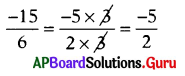(iv) Equivalent fraction to –$$\frac{2}{3}$$ = __________
Equivalent fraction to –$$\frac{2}{3}$$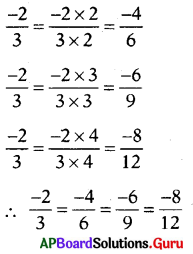Question 3.
Find the product:
(i) 2.1 × 6.3
2.1 × 6.3
= $$\frac{21}{10} \times \frac{63}{10}$$
= $$\frac{1323}{100}$$ = 13.23
∴ 2.1 × 6.3 = 13.23

(ii) 43.205 × 1.27
43.205 × 1.27
= $$\frac{43205}{1000} \times \frac{127}{100}$$
= $$\frac{43205 \times 127}{1000 \times 100}$$
= $$\frac{5487035}{100000}$$
∴ 43.205 × 1.27 = 54.87035

(iii) 7.641 × 3.5
7.64 1 × 3.5
= $$\frac{7641}{1000} \times \frac{35}{10}$$
= $$\frac{7641 \times 35}{1000 \times 10}$$
= $$\frac{267435}{10000}$$

∴ 7.641 × 3.5 = 26.7435.

(iv) 5.24 × 0.99
5.24 × 0.99
= $$\frac{524}{100} \times \frac{99}{100}$$
= $$\frac{524 \times 99}{100 \times 100}$$
= $$\frac{51876}{10000}$$

∴ 5.24 × 0.99 = 5.1876

Question 4.
Solve the following :
(i) 61.24 ÷ 0.4
61.24 ÷ 0.4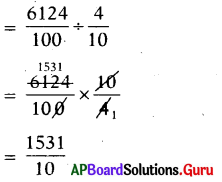∴ 61.24 ÷ 0.4 = 153.1

(ii) 23.45 ÷ 1.5
23.45 ÷ 1.5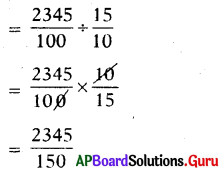∴ 23.45 ÷ 1.5 = 15.633….

(iii) 0.312 ÷ – 0.6
0.312 ÷ – 0.6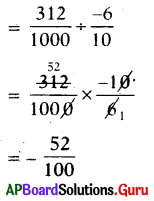∴ 0.312 ÷ – 0.6 = -0.52

(iv) 32.2 ÷ 2.2
32,2 ÷ 2.2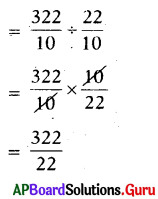∴ 32.2 ÷ 2.2 = 14.6363….,

Question 5.
Multiply 0.04 by – $$\frac{1}{2}$$
Given multiply 0.04 by –$$\frac{1}{2}$$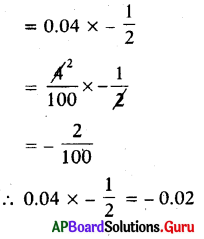Question 6.
Find standard form of – $$\frac{15}{35}$$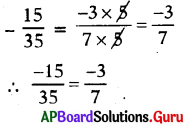Question 7.
A bus travelled 300 km In 7 $$\frac{1}{2}$$hours with uniform speed. Find how many km, it travelled in 1 hour.
Given distance travelled by bus in 7$$\frac{1}{2}$$
hours = 300 km

Distance travelled by bus in 1 hour
= 300 ÷ 7$$\frac{1}{2}$$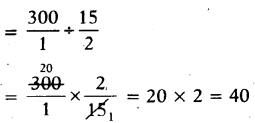Question 8.
Suvarna had 300. She spent $$\frac{1}{3}$$ of her money on notebooks and $$\frac{1}{4}$$ of the remaining on stationery items. How much money is left with ber?
Total money Suvarna had = ₹ 300
Money spent on notebooks
= $$\frac{1}{3}$$ of ₹ 300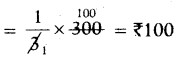Remaining amount
= Total money – spent on books
= 300 – 100 = ₹ 200

Money spent on stationery
= $$\frac{1}{4}$$ of remaining amount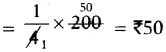Money left with Suvarna
= Total money – spent on books – spent on stationery
= 300 – 100 – 50
= 300 – 150
∴ Money left with Suvarna = ₹ 150

Question 9.
One litre of diesel costs ₹ 84.65. What is the cost of 12.5 liters of diesel?
Given cost of one litre diesel = ₹ 84.65
Cost of 12.5 liters diesel = 84.65 × 12.5
= $$\frac{8465}{100} \times \frac{125}{10}$$
= $$\frac{1058125}{1000}$$ = ₹ 1058.125
∴ Cost of 12.5 litres diesel = ₹ 1058.125Question 10.
Represent $$\frac{-2}{5}, \frac{-3}{5}, \frac{-1}{5}, \frac{3}{5}$$ on same number line.
Given fractions are $$\frac{-2}{5}, \frac{-3}{5}, \frac{-1}{5}, \frac{3}{5}$$
Ascending order: $$\frac{-3}{5}, \frac{-2}{5}, \frac{-1}{5}, \frac{3}{5}$$